# Colleges with the lowest SAT scores in Indiana

Top 10 colleges in Indiana with the lowest SAT scores
Looking for the colleges with the lowest SAT scores in Indiana? Well you're in luck! We've compiled a national college database and have created a list of the top 10 universities with the lowest SAT scores in Indiana below. If you are not a good test taker or worried about your test scores, this list is for you. These are the schools whose applicants had the lowest average SAT scores in Indiana, which means that you can get into these colleges with a lower SAT score. We also include each college's ACT scores and acceptance rate so that you can see where you would have the easiest time getting in. Read on to find out more.

## Indiana University Northwest SAT scores

The average SAT score for Indiana University Northwest is 1000.The average SAT score of 1000 breaks down into:

• SAT math: 500

The average ACT score for Indiana University Northwest is 20 and their acceptance rate is 79.6%.

## Anderson University SAT scores

The average SAT score for Anderson University is 1011.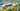The average SAT score of 1011 breaks down into:

• SAT math: 510

The average ACT score for Anderson University is 22 and their acceptance rate is 65.3%.

## Oakland City University SAT scores

The average SAT score for Oakland City University is 1016.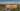The average SAT score of 1016 breaks down into:

• SAT math: 510

The average ACT score for Oakland City University is 19 and their acceptance rate is 49.7%.

## Indiana State University SAT scores

The average SAT score for Indiana State University is 1018.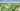The average SAT score of 1018 breaks down into:

• SAT math: 500

The average ACT score for Indiana State University is 20 and their acceptance rate is 89.9%.

## Indiana University East SAT scores

The average SAT score for Indiana University East is 1020.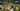The average SAT score of 1020 breaks down into:

• SAT math: 500

The average ACT score for Indiana University East is 20 and their acceptance rate is 64.9%.

## Indiana University South Bend SAT scores

The average SAT score for Indiana University South Bend is 1030.The average SAT score of 1030 breaks down into:

• SAT math: 510

The average ACT score for Indiana University South Bend is 20 and their acceptance rate is 81.9%.

## Indiana University Kokomo SAT scores

The average SAT score for Indiana University Kokomo is 1040.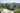The average SAT score of 1040 breaks down into:

• SAT math: 520

The average ACT score for Indiana University Kokomo is 21 and their acceptance rate is 80%.

## Bethel University Indiana SAT scores

The average SAT score for Bethel University Indiana is 1055.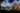The average SAT score of 1055 breaks down into:

• SAT math: 535

The average ACT score for Bethel University Indiana is 22 and their acceptance rate is 90%.

## Indiana University Southeast SAT scores

The average SAT score for Indiana University Southeast is 1060.The average SAT score of 1060 breaks down into:

• SAT math: 520

The average ACT score for Indiana University Southeast is 20 and their acceptance rate is 85.1%.

## Purdue University Calumet SAT scores

The average SAT score for Purdue University Calumet is 1060.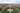The average SAT score of 1060 breaks down into:

• SAT math: 520